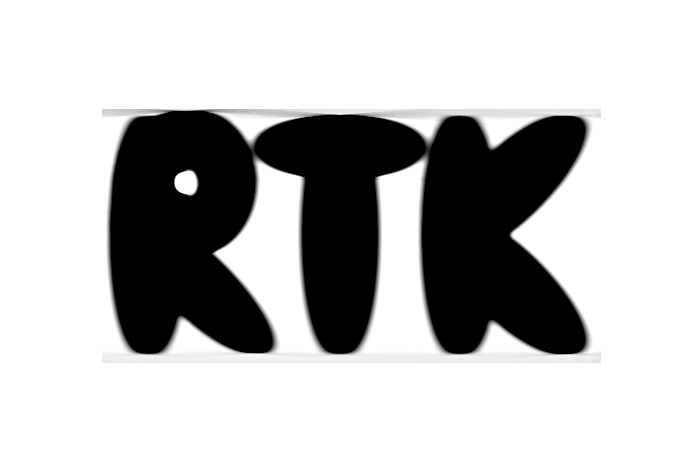RTK  2.1.0 Reconstruction Toolkit
rtk::RayConvexIntersectionImageFilter< TInputImage, TOutputImage > Class Template Reference

#include <rtkRayConvexIntersectionImageFilter.h>Inheritance diagram for rtk::RayConvexIntersectionImageFilter< TInputImage, TOutputImage >:Collaboration diagram for rtk::RayConvexIntersectionImageFilter< TInputImage, TOutputImage >:

## Public Types

using ConstPointer = itk::SmartPointer< const Self >

using ConvexShapePointer = ConvexShape::Pointer

using GeometryConstPointer = typename GeometryType::ConstPointer

using GeometryType = rtk::ThreeDCircularProjectionGeometry

using OutputImageRegionType = typename TOutputImage::RegionType

using Pointer = itk::SmartPointer< Self >

using PointType = ConvexShape::PointType

using ScalarType = ConvexShape::ScalarType

using Self = RayConvexIntersectionImageFilter

using Superclass = itk::InPlaceImageFilter< TInputImage, TOutputImage >

## Public Member Functions

virtual ::itk::LightObject::Pointer CreateAnother () const

virtual const char * GetNameOfClass () const

virtual ConvexShapeGetModifiableConvexShape ()

virtual const ConvexShapeGetConvexShape () const

virtual void SetConvexShape (ConvexShape *_arg)

virtual const GeometryTypeGetGeometry () const

virtual void SetGeometry (const GeometryType *_arg)

virtual double GetAttenuation ()

virtual void SetAttenuation (double _arg)

## Static Public Member Functions

static Pointer New ()

## Protected Member Functions

RayConvexIntersectionImageFilter ()

void VerifyPreconditions () ITKv5_CONST override

~RayConvexIntersectionImageFilter () override=default

## Private Attributes

double m_Attenuation = 0.

ConvexShapePointer m_ConvexShape

GeometryConstPointer m_Geometry

## Detailed Description

### template<class TInputImage, class TOutputImage> class rtk::RayConvexIntersectionImageFilter< TInputImage, TOutputImage >

Analytical projection of ConvexShape.

Test:
rtkfdktest.cxx, rtkforbildtest.cxx

Definition at line 40 of file rtkRayConvexIntersectionImageFilter.h.

## ◆ ConstPointer

template<class TInputImage , class TOutputImage >
 using rtk::RayConvexIntersectionImageFilter< TInputImage, TOutputImage >::ConstPointer = itk::SmartPointer

Definition at line 49 of file rtkRayConvexIntersectionImageFilter.h.

## ◆ ConvexShapePointer

template<class TInputImage , class TOutputImage >
 using rtk::RayConvexIntersectionImageFilter< TInputImage, TOutputImage >::ConvexShapePointer = ConvexShape::Pointer

Definition at line 55 of file rtkRayConvexIntersectionImageFilter.h.

## ◆ GeometryConstPointer

template<class TInputImage , class TOutputImage >
 using rtk::RayConvexIntersectionImageFilter< TInputImage, TOutputImage >::GeometryConstPointer = typename GeometryType::ConstPointer

Definition at line 54 of file rtkRayConvexIntersectionImageFilter.h.

## ◆ GeometryType

template<class TInputImage , class TOutputImage >
 using rtk::RayConvexIntersectionImageFilter< TInputImage, TOutputImage >::GeometryType = rtk::ThreeDCircularProjectionGeometry

Definition at line 53 of file rtkRayConvexIntersectionImageFilter.h.

## ◆ OutputImageRegionType

template<class TInputImage , class TOutputImage >
 using rtk::RayConvexIntersectionImageFilter< TInputImage, TOutputImage >::OutputImageRegionType = typename TOutputImage::RegionType

Convenient type alias.

Definition at line 52 of file rtkRayConvexIntersectionImageFilter.h.

## ◆ Pointer

template<class TInputImage , class TOutputImage >
 using rtk::RayConvexIntersectionImageFilter< TInputImage, TOutputImage >::Pointer = itk::SmartPointer

Definition at line 48 of file rtkRayConvexIntersectionImageFilter.h.

## ◆ PointType

template<class TInputImage , class TOutputImage >
 using rtk::RayConvexIntersectionImageFilter< TInputImage, TOutputImage >::PointType = ConvexShape::PointType

Definition at line 57 of file rtkRayConvexIntersectionImageFilter.h.

## ◆ ScalarType

template<class TInputImage , class TOutputImage >
 using rtk::RayConvexIntersectionImageFilter< TInputImage, TOutputImage >::ScalarType = ConvexShape::ScalarType

Definition at line 56 of file rtkRayConvexIntersectionImageFilter.h.

## ◆ Self

template<class TInputImage , class TOutputImage >
 using rtk::RayConvexIntersectionImageFilter< TInputImage, TOutputImage >::Self = RayConvexIntersectionImageFilter

Standard class type alias.

Definition at line 46 of file rtkRayConvexIntersectionImageFilter.h.

## ◆ Superclass

template<class TInputImage , class TOutputImage >
 using rtk::RayConvexIntersectionImageFilter< TInputImage, TOutputImage >::Superclass = itk::InPlaceImageFilter

Definition at line 47 of file rtkRayConvexIntersectionImageFilter.h.

## ◆ RayConvexIntersectionImageFilter()

template<class TInputImage , class TOutputImage >
 rtk::RayConvexIntersectionImageFilter< TInputImage, TOutputImage >::RayConvexIntersectionImageFilter ( )
protected

## ◆ ~RayConvexIntersectionImageFilter()

template<class TInputImage , class TOutputImage >
 rtk::RayConvexIntersectionImageFilter< TInputImage, TOutputImage >::~RayConvexIntersectionImageFilter ( )
overrideprotecteddefault

## Member Function Documentation

template<class TInputImage , class TOutputImage >
 void rtk::RayConvexIntersectionImageFilter< TInputImage, TOutputImage >::BeforeThreadedGenerateData ( )
overrideprotectedvirtual

ConvexShape must be created in the BeforeThreadedGenerateData in the daugter classes.

Reimplemented from itk::ImageSource< TOutputImage >.

## ◆ CreateAnother()

template<class TInputImage , class TOutputImage >
 virtual::itk::LightObject::Pointer rtk::RayConvexIntersectionImageFilter< TInputImage, TOutputImage >::CreateAnother ( ) const
virtual

Reimplemented from itk::Object.

template<class TInputImage , class TOutputImage >
overrideprotectedvirtual

Apply changes to the input image requested region.

Reimplemented from itk::ImageSource< TOutputImage >.

## ◆ GetAttenuation()

template<class TInputImage , class TOutputImage >
 virtual double rtk::RayConvexIntersectionImageFilter< TInputImage, TOutputImage >::GetAttenuation ( )
virtual

Get / Set the attenuation $$\mu$$ to simulate the attenuated line integral. Default is 0. The attenuated line integral model is

$p=\int f(\underline s+r\underline{d})\exp(\mu r)\mathrm{d}r$

with $$\underline s$$ and $$\underline d$$ the ray source and direction. The value is therefore:

\begin{eqnarray*} p&=&\int_{n}^{f} d\exp(\mu r)\mathrm{d}r\\ &=&\dfrac{d}{\mu}\left(\exp(\mu {f})-\exp(\mu n)\right) \end{eqnarray*}

with $$n$$ and $$f$$ the distances from source to intersection points near and far from the source and $$d$$ m_Density.

## ◆ GetConvexShape()

template<class TInputImage , class TOutputImage >
 virtual const ConvexShape* rtk::RayConvexIntersectionImageFilter< TInputImage, TOutputImage >::GetConvexShape ( ) const
virtual

Get / Set the object pointer to the ConvexShape.

## ◆ GetGeometry()

template<class TInputImage , class TOutputImage >
 virtual const GeometryType* rtk::RayConvexIntersectionImageFilter< TInputImage, TOutputImage >::GetGeometry ( ) const
virtual

Get / Set the object pointer to projection geometry

## ◆ GetModifiableConvexShape()

template<class TInputImage , class TOutputImage >
 virtual ConvexShape* rtk::RayConvexIntersectionImageFilter< TInputImage, TOutputImage >::GetModifiableConvexShape ( )
virtual

Get / Set the object pointer to the ConvexShape.

## ◆ GetNameOfClass()

template<class TInputImage , class TOutputImage >
 virtual const char* rtk::RayConvexIntersectionImageFilter< TInputImage, TOutputImage >::GetNameOfClass ( ) const
virtual

Run-time type information (and related methods).

Reimplemented from itk::InPlaceImageFilter< TInputImage, TOutputImage >.

## ◆ New()

template<class TInputImage , class TOutputImage >
 static Pointer rtk::RayConvexIntersectionImageFilter< TInputImage, TOutputImage >::New ( )
static

Method for creation through the object factory.

## ◆ SetAttenuation()

template<class TInputImage , class TOutputImage >
 virtual void rtk::RayConvexIntersectionImageFilter< TInputImage, TOutputImage >::SetAttenuation ( double _arg )
virtual

Get / Set the attenuation $$\mu$$ to simulate the attenuated line integral. Default is 0. The attenuated line integral model is

$p=\int f(\underline s+r\underline{d})\exp(\mu r)\mathrm{d}r$

with $$\underline s$$ and $$\underline d$$ the ray source and direction. The value is therefore:

\begin{eqnarray*} p&=&\int_{n}^{f} d\exp(\mu r)\mathrm{d}r\\ &=&\dfrac{d}{\mu}\left(\exp(\mu {f})-\exp(\mu n)\right) \end{eqnarray*}

with $$n$$ and $$f$$ the distances from source to intersection points near and far from the source and $$d$$ m_Density.

## ◆ SetConvexShape()

template<class TInputImage , class TOutputImage >
 virtual void rtk::RayConvexIntersectionImageFilter< TInputImage, TOutputImage >::SetConvexShape ( ConvexShape * _arg )
virtual

Get / Set the object pointer to the ConvexShape.

## ◆ SetGeometry()

template<class TInputImage , class TOutputImage >
 virtual void rtk::RayConvexIntersectionImageFilter< TInputImage, TOutputImage >::SetGeometry ( const GeometryType * _arg )
virtual

Get / Set the object pointer to projection geometry

## ◆ VerifyPreconditions()

template<class TInputImage , class TOutputImage >
 void rtk::RayConvexIntersectionImageFilter< TInputImage, TOutputImage >::VerifyPreconditions ( )
overrideprotectedvirtual

Checks that inputs are correctly set.

Reimplemented from itk::ProcessObject.

## ◆ m_Attenuation

template<class TInputImage , class TOutputImage >
 double rtk::RayConvexIntersectionImageFilter< TInputImage, TOutputImage >::m_Attenuation = 0.
private

Definition at line 112 of file rtkRayConvexIntersectionImageFilter.h.

## ◆ m_ConvexShape

template<class TInputImage , class TOutputImage >
 ConvexShapePointer rtk::RayConvexIntersectionImageFilter< TInputImage, TOutputImage >::m_ConvexShape
private

Definition at line 110 of file rtkRayConvexIntersectionImageFilter.h.

## ◆ m_Geometry

template<class TInputImage , class TOutputImage >
 GeometryConstPointer rtk::RayConvexIntersectionImageFilter< TInputImage, TOutputImage >::m_Geometry
private

Definition at line 111 of file rtkRayConvexIntersectionImageFilter.h.

The documentation for this class was generated from the following file: